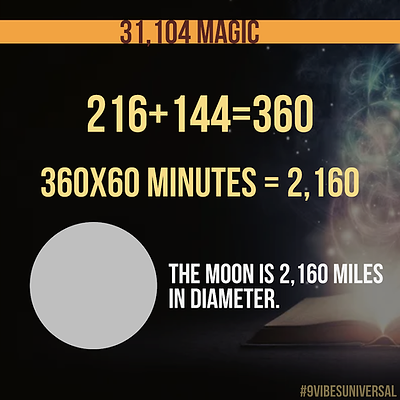top of page# 31,104 (144)

First we must discuss 144

144,000 petals in the crown chakra

All five platonic solids = 14,400.

12th Fibonacci number = 144

24 he x 60 = 1,440 min

1,440 x 60 = 86,400 seconds

86,400/21,600= 4 seconds
= 1 breath

1,440 octahedron

The number 144 is used in the Bible only once.

144,000 outer casing stones on the pyramid of Giza.

First 144 digits of π after the decimal sum to 666.

144,000 days is equal to 400 years using the old calendar of 360 days.

9x16=144
72x2=144
12x12=144
2,160/15=144
72^2+244^2=25,920
144x2=288
864/6=144
864/3=288

According to Plato there is great change every 144 years.
There is a great change in humanity every 1,728 years.## 31,104 (216)

2,160 orbits of Jupiter.

Maya long count is 2,160 orbits of Jupiter and 144 solar cycles.

Earth nautical mile 21,600.
Moon is 2,160 miles in diameter.

216 hours in 9 days
4,536 min is 3.15 days.
3.15 days is equal to 3 days and 216 min.

216th number in the Fibonacci sequence is 216.

Pythagoras 3-4-5 triangle
= 216

216x144=31,104
216+144=360

6x6x6=216
3^3+4^3+5^3=6^3 = 216

216x2=432
72x3=216
10,800x2= 21,600
360x60=21,600
2,160x4=8,640
69xπ=216
2,160/5=432
2,160/6=360
864/4=216
720x3=2,160
108x2=216
36x6=216
2,160x2=4,320
25,920-4,320=21,600

Exodus 19,20,21
Each being 72 letters long
72x3=216

216^3= is the smallest cube

432+234=666## 31,104 (Bible)

There are 31,104 verses in the Old New King James version of the Bible.## 31,104 (Bible part 2)

12 zodiac's
2,160 days per zodiac
2,160x12=25,920
25,920x12,000=311,040,000

12 tribes
12,000 per tribe
12,000x12=144,000

311,040,000÷144,000=2,160

From astrology to the Bible.
From the Bible to astrology.

Amazing!## 31,104 (Speed of light, Time and Pyramids)

Speed of Light 186,624 miles per second
86,400 seconds in a day
Pyramid of Giza slope = 51.84°

186,624 x 86,400 = 16,124,313,600

Now let’s apply the Pyramid slope
16,124,313,600 ÷ 5184 = 3,110,400

There's that number again!